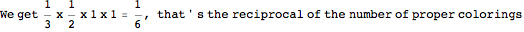Cavity method to count colorings

Sometimes we have an easy way to find marginal probabilities of spin configurations, cavity method gives us a way to combine those marginal probabilities to get a partition functon.

For example, consider all possible ways of coloring a kite graph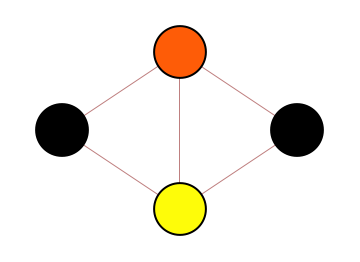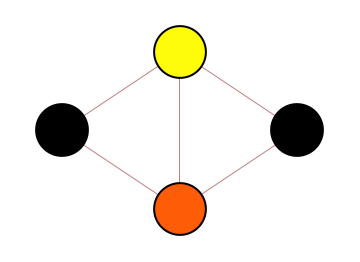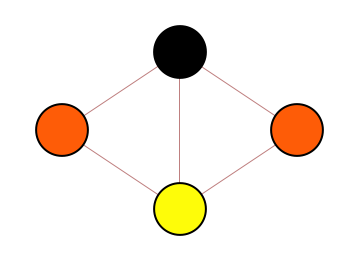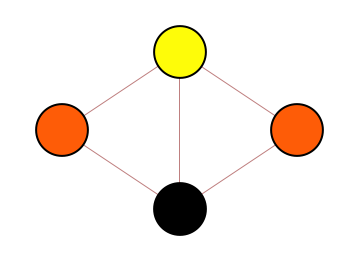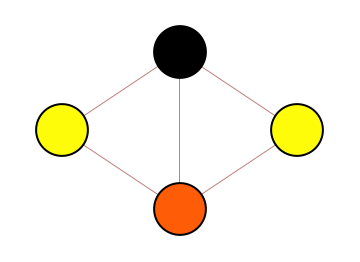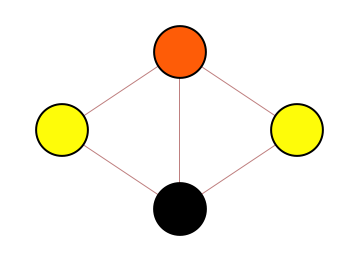Taking all configurations to be equally likely, we can see from this that the probability of the leftmost node taking black color is 1/3. In the graph above, this can be found by counting all configurations, however more efficient methods exist to find this marginal probability. The question is now whether we can combine such probabilities to find the total number of configurations.

Step 0 : pick some arbitrary order over vertices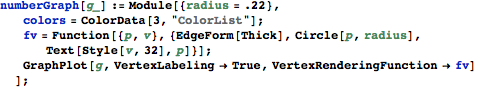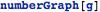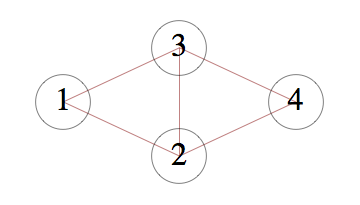Step 1 : pick an arbitrary proper coloring using greedy methodStep 2 : suppose proper colorings are sampled uniformly, find the probability of first vertex assuming the color picked for it in Step 1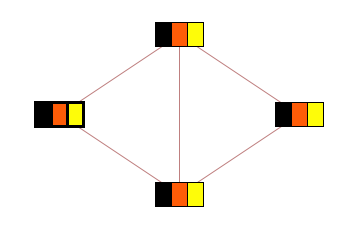By symmetry, this value is 1/3

Step 3 : remove this vertex, and remove it's color from the list of allowed colors for adjacent vertices.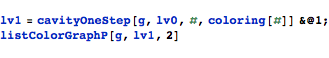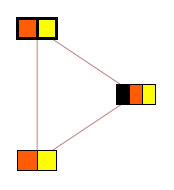Step 4 : find probability of the next node taking it' s assigned color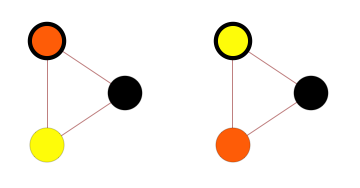By symmetry you can see this value is 1/2

Step 5 : repeat steps 3 and 4 for the next vertex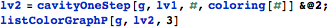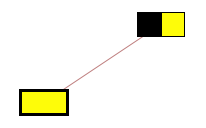the probability of node assuming it' s chosen color is 1

Step 6: repeat steps 3 and 4 again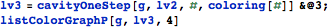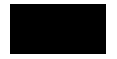Again the probability of remaining node taking black is 1

Step 7: multiply together the marginal probabilities